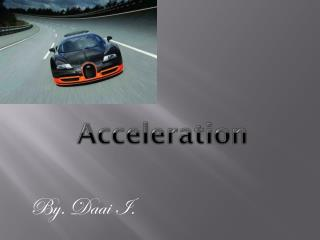DownloadDownload PresentationAcceleration

# Acceleration

Télécharger la présentation## Acceleration

- - - - - - - - - - - - - - - - - - - - - - - - - - - E N D - - - - - - - - - - - - - - - - - - - - - - - - - - -
##### Presentation Transcript

1. Acceleration By. DaaiI.

2. Acceleration and motion • Acceleration is the change in velocity divided by the time it takes for the change to occur. • When you watch a plane moving for the first few seconds it seems to barley move, however with each passing second you can see it moving faster and faster , this is an example of acceleration.

3. Acceleration • Like velocity, acceleration has a direction. If an object speeds up, the acceleration is in the direction that the object is moving. • If an object slows down, the acceleration is opposite to the direction that the object is moving.

4. Types of accelerating • There are three types of acceleration ,speeding up, slowing down, and changing direction . • Speeding up is like when you get on a bike and begin to pedal. The bike moves slowly at first, and then accelerates because its speed increases. • Slowing down is like when you are riding a bike at a quick speed, and you apply the brakes. This causes you to slow down. • Changing direction in acceleration is that the motion ,is not always along a straight line.

5. Calculating Acceleration • If an object is moving in a straight line, its acceleration can be calculated using this equation.

6. Positive and Negative Acceleration • Positive acceleration is when the object is speeding up, but negative acceleration ,is when the object is slowing down. • When you speed up, your final speed always will be greater than your initial speed. So subtracting your initial speed from your final speed gives a positive number. • Because your final speed is less than your initial speed, your acceleration is negative when you slow down.

7. Graphing acceleration • A speed-time graph can be used to find acceleration. When the line rises, the object is speeding up. • When the line falls, the object is slowing down.

8. I hope you enjoyed Thank you